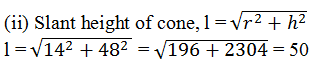Guru

# The volume of a right circular cone is 9856cm3. If the diameter of the base is 28cm, find (i) height of the cone (ii) slant height of the cone (iii) curved surface area of the cone (Assume π = 22/7) Q.6

• 0

Please guide me the best way for solving the question of class 9th math of Surface areas and Volumes chapter of exercise 13.7  of math of question no.6 What is the best way for solving this question, please guide me The volume of a right circular cone is 9856cm3. If the diameter of the base is 28cm, find (i) height of the cone (ii) slant height of the cone (iii) curved surface area of the cone (Assume π = 22/7)

Share

1. Volume of a right circular cone = 9856 cm3

Diameter of the base = 28 cm

(i) Radius of cone, r = (28/2) cm = 14 cm

Let the height of the cone be h

Volume of cone, V = (1/3) πr2h

(1/3) πr2h = 9856

(1/3)×(22/7) ×14×14×h = 9856

h = 48

The height of the cone is 48 cm.Slant height of the cone is 50 cm.

(iii) curved surface area of cone = πrl

= (22/7)×14×50

= 2200

curved surface area of the cone is 2200 cm2.

• 0